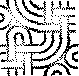#The Evolution Ofa Haskell Programmer

I think of this as the ObfuscatedPerl contest meets MathematicsMadeDifficult?. The later versions are all based on ludicrously overpowered excursions into CategoryTheory.

Somebody in OnMonads pointed out that the above document doesn't have a monadic factorial, so I will provide one. One imagines that this would be written by someone who came from an asm background...

```    slice :: [a] -> Int -> Int -> [a]
slice _ _ 1 = []
slice x:xs 0 end = x : (slice xs 0 (end - 1))
slice x:xs beg end = slice xs (beg - 1) (end - 1)

data Variable = Var Int
data Scope    = [Int]
data ScopeFn? a = Scope -> (a, Scope) deriving Monad;
new :: ScopeFn? Variable
new scope fn = fn ((Var (length scope)), scope)
set :: Variable -> Int -> ScopeFn? ()
set (Var x) value scope fn = fn ((), ((slice scope 0 x) ++ [value] ++ (slice scope (x + 2) (length scope))))
get :: Variable -> ScopeFn? Int
get (Var x) scope fn = fn (scope !! x, scope)

(>>=) :: ScopeFn? a -> (a -> ScopeFn? b) -> ScopeFn? b
(>>=) fn1 fn2 scope1 =
let (value, scope2) = fn1 scope1
in  fn2 value scope2
return :: a -> ScopeFn? a
return x scope = (x, scope)

prod :: Variable -> Variable -> Variable -> ScopeFn? ()
prod res a b = do
a' = get a
b' = get b
set res (a' * b')
dec  :: Variable -> ScopeFn? ()
dec v = do
v' = get v
set v (v' - 1)
inScope :: ScopeFn? a -> a
inScope fn =
ret where (ret, _) = fn []

fac :: Int -> Int
fac' x y = do
prod x x y
dec y
y' <- get y
if (y' == 0)
then return ()
else fac' x y

fac x = inScope do
total <- new
va <- new
set va x
set total 1
fac' total va
ret <- get va
return va

```EditText of this page (last edited May 4, 2012) or FindPage with title or text search